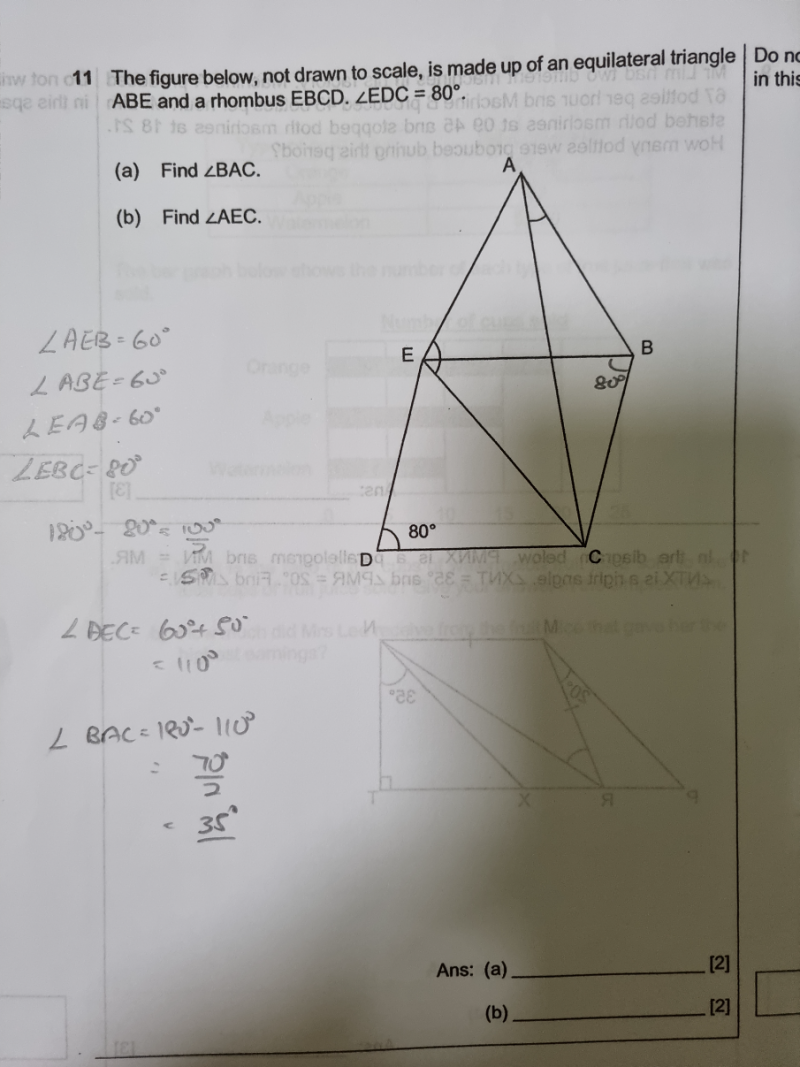# QuestionIs my steps correct?thank you.

Your steps are incorrect.  Also, remember never write statements like this:

180-80 = 100/2 as they are NOT EQUAL.  It is a common mistake students make to save time by combining 2 mathematical statements into one.  It should be 180-80 = 100, then 100/2 = 50  or (180-80)/2 = 50 if you want to combine steps.

Solution:

(a) Since AEB is an equilateral triangle, AE = AB = EB

and since EBCD is a rhombus, EB = BC = CD = DE

Angle EBC = Angle EDC = 80  (Opp angles inside a rhombus)

Angle ABE = 60 (Angles inside equilateral triangle)

Angle ABC = 60 + 80 = 140

Hence Angle BAC =  (180-140) / 2 = 200  (angles in an isosceles triangle, AB=BC)

(b)   Angle BEC = (180-80)/2 = 100  (angles in an isosceles triangle, EB = BC)

Angle AEB = 60 (Angles inside equilateral triangle)

Hence Angle AEC = 60 + 100 = 1600

PS: I present the solution comprehensively for your understanding how to derive the answer.  However once you have mastered it, the solution for (a)  can be shortened to this:

(a) Angle EBC = Angle EDC = 80,  Angle ABE = 60

Angle ABC = 60 + 80 = 140

Hence Angle BAC =  (180-140) / 2 = 200